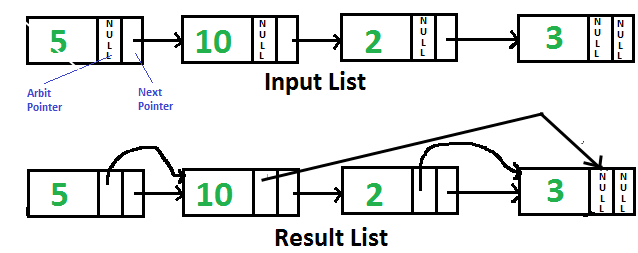# Cpp Algorithm-Rearrange a Linked List in Zig-Zag fashionCpp Algorithm-Rearrange a Linked List in Zig-Zag fashion-Linked list Given a linked list, rearrange it such that converted list should be

Given a linked list, rearrange it such that converted list should be of the form a < b > c < d > e < f .. where a, b, c.. are consecutive data node of linked list. Examples :

```Input:  1->2->3->4
Output: 1->3->2->4

Input:  11->15->20->5->10
Output: 11->20->5->15->10

```

A simple approach to do this, is to sort the linked list using merge sort and then swap alternate, but that requires O(n Log n) time complexity. Here n is number of elements in linked list.

An efficient approach which requires O(n) time is, using a single scan similar to bubble sort and then maintain a flag for representing which order (< or >) currently we are. If the current two elements are not in that order then swap those elements otherwise not. Please refer this for detailed explanation of swapping order.

C++ programming:

``````// C++ program to arrange linked list in zigzag fashion
#include <bits/stdc++.h>
using namespace std;

struct Node
{
int data;
struct Node* next;
};

// This function distributes the Node in zigzag fashion
{
// If flag is true, then next node should be greater
// in the desired output.
bool flag = true;

while (current->next != NULL)
{
if (flag)  /* "<" relation expected */
{
/* If we have a situation like A > B > C
where A, B and C are consecutive Nodes
in list we get A > B < C by swapping B
and C */
if (current->data > current->next->data)
swap(current->data, current->next->data);
}
else /* ">" relation expected */
{
/* If we have a situation like A < B < C where
A, B and C  are consecutive Nodes in list we
get A < C > B by swapping B and C */
if (current->data < current->next->data)
swap(current->data, current->next->data);
}

current = current->next;
flag = !flag;  /* flip flag for reverse checking */
}
}

/* UTILITY FUNCTIONS */
/* Function to push a Node */
{
/* allocate Node */
struct Node* new_Node = new Node;

/* put in the data  */
new_Node->data  = new_data;

/* link the old list off the new Node */

/* move the head to point to the new Node */
}

/* Function to print linked list */
void printList(struct Node *Node)
{
while (Node != NULL)
{
printf("%d->", Node->data);
Node = Node->next;
}
printf("NULL");
}

/* Drier program to test above function*/
int main(void)
{

// create a list 4 -> 3 -> 7 -> 8 -> 6 -> 2 -> 1
// answer should be -> 3  7  4  8  2  6  1

return (0);
}``````

Output :

```Given linked list
4->3->7->8->6->2->1->NULL

3->7->4->8->2->6->1->NULL```

In above code, push function pushes the node at the front of the linked list, the code can be easily modified for pushing node at the end of list. Other thing to note is, swapping of data between two nodes is done by swap by value not swap by links for simplicity, for swap by links technique please see this.

READ  C Algorithm - Move last element to front of a given Linked List

Time complexity : O(n)
Auxiliary Space : O(1)#### Venkatesan Prabu

Wikitechy Founder, Author, International Speaker, and Job Consultant. My role as the CEO of Wikitechy, I help businesses build their next generation digital platforms and help with their product innovation and growth strategy. I'm a frequent speaker at tech conferences and events.

X